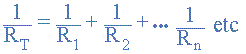(347) 552-2258 help@rossmanngroup.com

# resistor goes from 1MΩ to 0.5MΩ??

#### JorgePayar

##### Member
Hi, I have replaced a 1MΩ resistor in a 820 2850 and I measure it before 1MΩ when I solder it 0.5MΩ
The resistor is r6995 and I have the same issue with r6996
I didn´t apply too much heat so what can be?

Last edited:

#### Crizz

##### Member
This is ohms LawIf you have R1 and R2 in series you can calculate the total resistance R[SUB]T[/SUB]=R1+R2
Example:
R1=1MΩ
R2=2MΩ

==> R[SUB]T[/SUB]=1MΩ+2MΩ=3MΩ

BUT if you have Resistors in parallel you have to do this calculation:Because there is another resistor in parallel in your case the U6990, the R[SUB]T[/SUB] will always be less than 1MΩ!
One important point to remember about resistors in parallel, is that the total circuit resistance of any two resistors connected together in parallel will always be LESS than the value of the smallest resistor in that combination.
You can test it if you unsolder R6995 and measure the resistance of the pads. You will get the value of U6990 witch has to be over 0.5MΩ but less than 1MΩ#### Attachments

• 1.7 KB Views: 0
Last edited:

#### JorgePayar

##### Member
Cool!! Im learning so much in this forum!!
Thanks for taking the time to reply Crizz.